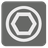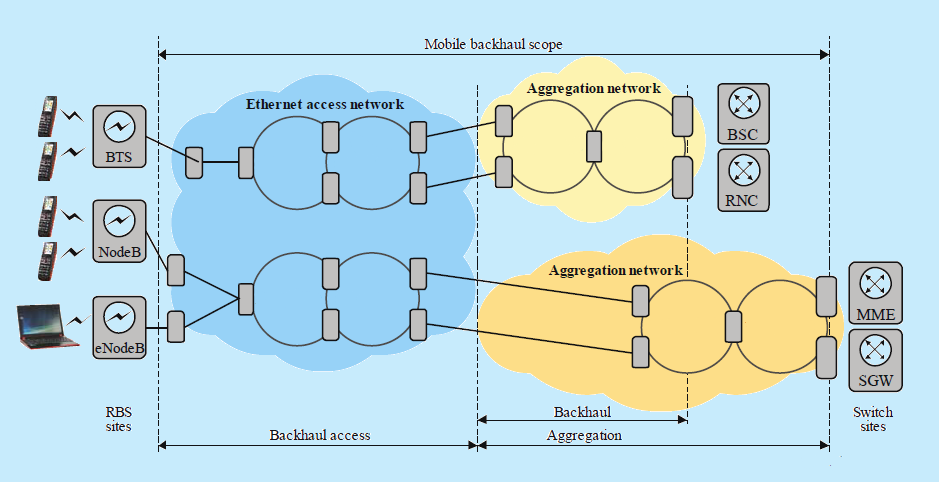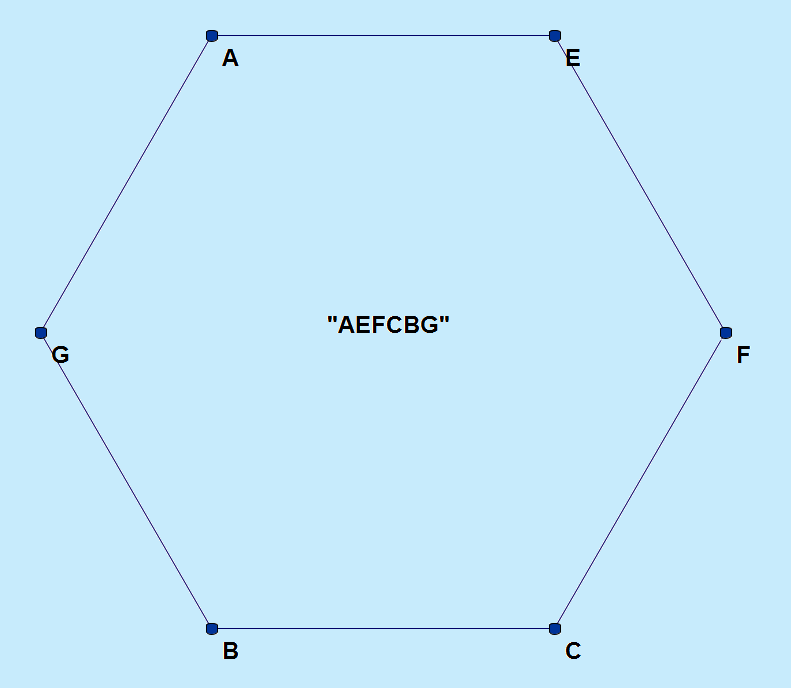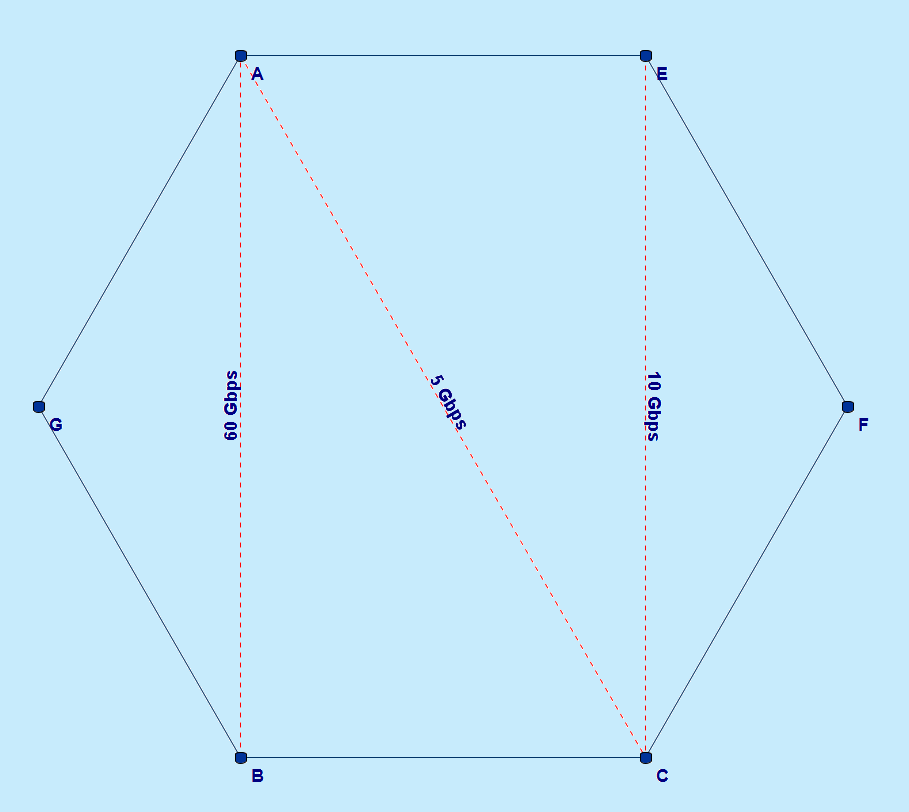Ethernet Ring Dimensioning
Simple
English

A ring topology is a network configuration where the devices are connected to each other in a circular shape. Optical rings are widely used in mobile networks to transport the traffic from a base station to the backbone, through the mobile backhaul.### Definition of a ring

For the sake of simplicity, in this task, we define a ring as a connex graph which vertexes:

1. - have a degree 2, which means they have 2 incident edges.
2. - are connected to two distinct vertexes

These two conditions ensure that there is a single link between each pair of adjacent nodes. Ring are represented as a string made of distinct characters, which position in the string reflects the position in the ring. For instance, the ring "AEFCBG" defines the following topology:### Shortest path asymmetric routing

Multiple traffic flows will be routed on the ring.
Each flow is routed on the shortest path from the ingress node (starting point of the traffic) to the egress node (exit point of the traffic).
If there are two shortest paths, the path which order fits the ring definition is kept.
Let's consider the following situation:The path from A to B (and from B to A) will be "A - G - B", because it uses only two links, while the path going the other way around ("A - E - F - C - B") uses five links.
However, there are two paths of equal length to route a traffic from A to C: "A - E - F - C" and "A - G - B - C". The first one, "A - E - F - C", is kept: it is the same order as in the ring definition ("AEFCBG").
The traffic from C to A will use the path "C - B - G - A": the routing is asymmetric in that case.

### Flow aggregation

A traffic flow is represented as a couple (s, dr) where:

1. - s is a string of length 2, containing the ingress node and the egress node.
2. - dr is the data rate in Gbps (gigabit per second).

The data rate of a traffic flow will be counted for all the links of the shortest path.
A traffic flow ("AB", 5) means that 5 Gbps will be routed on the shortest path from A to B.
We count 5 Gbps on the link "AG" and 5 Gbps on the link "GB". For a traffic flow ("CA", 15), we count 15 Gbps on the links "CB", "BG", and "GA".
In order to simplify the model, we consider that the traffic flows ingress -> egress and egress -> ingress are equivalent with regard to dimensioning.
Two traffic flows ("AG", 3) and ("GA", 3) induce a 6-Gbps flow on the link AG, as would ("AG", 6) or ("GA", 6): links are not directional. Given a list of traffic flows, we consider the resulting bandwidth on each link to dimension the ring.